### Home > MC2 > Chapter Ch11 > Lesson 11.2.3 > Problem11-56

11-56.
1. Simplify each numerical expression. Homework Help ✎

1.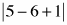2.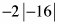3.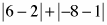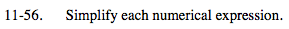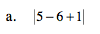Simplify within the absolute value signs.

What is 5 − 6 +1?

|5 − 6 +1| = |0|

The absolute value of 0 is 0.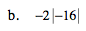First find the absolute value of −16.

The absolute value is the distance a number is away from 0, and is always a non negative number.
Since −16 is 16 units away from zero, the absolute value is 16.

What is the product of −2(16)?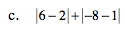Simplify within the absolute value signs first.

6 − 2 = 4 and −8 − 1 = −9.
Therefore we have: |4| + |−9|
What is the absolute value for each of these numbers?

4 + 9 = 13